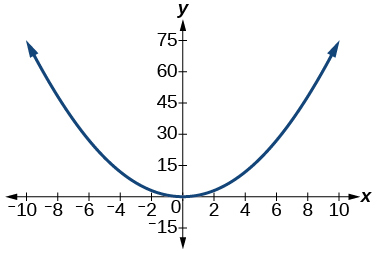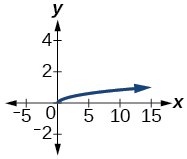# 5.8 Modeling using variation  (Page 5/14)

 Page 5 / 14

$\text{\hspace{0.17em}}y\text{\hspace{0.17em}}$ varies jointly as the square of $\text{\hspace{0.17em}}x\text{\hspace{0.17em}}$ and of $\text{\hspace{0.17em}}z\text{\hspace{0.17em}}$ and inversely as the square root of $\text{\hspace{0.17em}}w\text{\hspace{0.17em}}$ and of $\text{\hspace{0.17em}}t\text{\hspace{0.17em}.}$ When $\text{\hspace{0.17em}}x=2,\text{\hspace{0.17em}}$ $z=3,\text{\hspace{0.17em}}$ $w=16,\text{\hspace{0.17em}}$ and $\text{\hspace{0.17em}}t=3,\text{\hspace{0.17em}}$ then $\text{\hspace{0.17em}}y=1.\text{\hspace{0.17em}}$ Find $\text{\hspace{0.17em}}y\text{\hspace{0.17em}}$ when $\text{\hspace{0.17em}}x=3,\text{\hspace{0.17em}}$ $z=2,\text{\hspace{0.17em}}$ and $\text{\hspace{0.17em}}t=5.$

## Technology

For the following exercises, use a calculator to graph the equation implied by the given variation.

$\text{\hspace{0.17em}}y\text{\hspace{0.17em}}$ varies directly with the square of $\text{\hspace{0.17em}}x\text{\hspace{0.17em}}$ and when

$y=\frac{3}{4}{x}^{2}$$\text{\hspace{0.17em}}y\text{\hspace{0.17em}}$ varies directly as the cube of $\text{\hspace{0.17em}}x\text{\hspace{0.17em}}$ and when

$\text{\hspace{0.17em}}y\text{\hspace{0.17em}}$ varies directly as the square root of $\text{\hspace{0.17em}}x\text{\hspace{0.17em}}$ and when

$y=\frac{1}{3}\sqrt{x}$$\text{\hspace{0.17em}}y\text{\hspace{0.17em}}$ varies inversely with $\text{\hspace{0.17em}}x\text{\hspace{0.17em}}$ and when

$\text{\hspace{0.17em}}y\text{\hspace{0.17em}}$ varies inversely as the square of $\text{\hspace{0.17em}}x\text{\hspace{0.17em}}$ and when

$y=\frac{4}{{x}^{2}}$## Extensions

For the following exercises, use Kepler’s Law, which states that the square of the time, $\text{\hspace{0.17em}}T,\text{\hspace{0.17em}}$ required for a planet to orbit the Sun varies directly with the cube of the mean distance, $\text{\hspace{0.17em}}a,\text{\hspace{0.17em}}$ that the planet is from the Sun.

Using Earth’s time of 1 year and mean distance of 93 million miles, find the equation relating $\text{\hspace{0.17em}}T\text{\hspace{0.17em}}$ and $\text{\hspace{0.17em}}a.\text{\hspace{0.17em}}$

Use the result from the previous exercise to determine the time required for Mars to orbit the Sun if its mean distance is 142 million miles.

1.89 years

Using Earth’s distance of 150 million kilometers, find the equation relating $\text{\hspace{0.17em}}T\text{\hspace{0.17em}}$ and $\text{\hspace{0.17em}}a.$

Use the result from the previous exercise to determine the time required for Venus to orbit the Sun if its mean distance is 108 million kilometers.

0.61 years

Using Earth’s distance of 1 astronomical unit (A.U.), determine the time for Saturn to orbit the Sun if its mean distance is 9.54 A.U.

## Real-world applications

For the following exercises, use the given information to answer the questions.

The distance $\text{\hspace{0.17em}}s\text{\hspace{0.17em}}$ that an object falls varies directly with the square of the time, $\text{\hspace{0.17em}}t,\text{\hspace{0.17em}}$ of the fall. If an object falls 16 feet in one se c ond, how long for it to fall 144 feet?

3 seconds

The velocity $\text{\hspace{0.17em}}v\text{\hspace{0.17em}}$ of a falling object varies directly to the time, $\text{\hspace{0.17em}}t,\text{\hspace{0.17em}}$ of the fall. If after 2 seconds, the velocity of the object is 64 feet per second, what is the velocity after 5 seconds?

The rate of vibration of a string under constant tension varies inversely with the length of the string. If a string is 24 inches long and vibrates 128 times per second, what is the length of a string that vibrates 64 times per second?

48 inches

The volume of a gas held at constant temperature varies indirectly as the pressure of the gas. If the volume of a gas is 1200 cubic centimeters when the pressure is 200 millimeters of mercury, what is the volume when the pressure is 300 millimeters of mercury?

The weight of an object above the surface of Earth varies inversely with the square of the distance from the center of Earth. If a body weighs 50 pounds when it is 3960 miles from Earth’s center, what would it weigh it were 3970 miles from Earth’s center?

49.75 pounds

The intensity of light measured in foot-candles varies inversely with the square of the distance from the light source. Suppose the intensity of a light bulb is 0.08 foot-candles at a distance of 3 meters. Find the intensity level at 8 meters.

The current in a circuit varies inversely with its resistance measured in ohms. When the current in a circuit is 40 amperes, the resistance is 10 ohms. Find the current if the resistance is 12 ohms.

33.33 amperes

the third and the seventh terms of a G.P are 81 and 16, find the first and fifth terms.
if a=3, b =4 and c=5 find the six trigonometric value sin
pls how do I factorize x⁴+x³-7x²-x+6=0
in a function the input value is called
how do I test for values on the number line
if a=4 b=4 then a+b=
a+b+2ab
Kin
commulative principle
a+b= 4+4=8
Mimi
If a=4 and b=4 then we add the value of a and b i.e a+b=4+4=8.
Tariq
what are examples of natural number
an equation for the line that goes through the point (-1,12) and has a slope of 2,3
3y=-9x+25
Ishaq
show that the set of natural numberdoes not from agroup with addition or multiplication butit forms aseni group with respect toaaddition as well as multiplication
x^20+x^15+x^10+x^5/x^2+1
evaluate each algebraic expression. 2x+×_2 if ×=5
if the ratio of the root of ax+bx+c =0, show that (m+1)^2 ac =b^2m
By the definition, is such that 0!=1.why?
(1+cosA+IsinA)(1+cosB+isinB)/(cos@+isin@)(cos$+isin$)
hatdog
Mark
jaks
Ryan
how we can draw three triangles of distinctly different shapes. All the angles will be cutt off each triangle and placed side by side with vertices touching

#### Get Jobilize Job Search Mobile App in your pocket Now!ByByBy RhodesBy Steve GibbsBy John GabrieliBy OpenStaxBy Sandhills MLTBy Edgar DelgadoBy OpenStaxBy Hope PercleBy OpenStaxBy Ryan Lowe